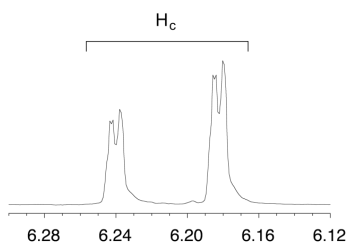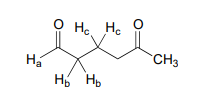# 9.9: Complex Coupling in Proton Spectra

$$\newcommand{\vecs}{\overset { \rightharpoonup} {\mathbf{#1}} }$$ $$\newcommand{\vecd}{\overset{-\!-\!\rightharpoonup}{\vphantom{a}\smash {#1}}}$$$$\newcommand{\id}{\mathrm{id}}$$ $$\newcommand{\Span}{\mathrm{span}}$$ $$\newcommand{\kernel}{\mathrm{null}\,}$$ $$\newcommand{\range}{\mathrm{range}\,}$$ $$\newcommand{\RealPart}{\mathrm{Re}}$$ $$\newcommand{\ImaginaryPart}{\mathrm{Im}}$$ $$\newcommand{\Argument}{\mathrm{Arg}}$$ $$\newcommand{\norm}{\| #1 \|}$$ $$\newcommand{\inner}{\langle #1, #2 \rangle}$$ $$\newcommand{\Span}{\mathrm{span}}$$ $$\newcommand{\id}{\mathrm{id}}$$ $$\newcommand{\Span}{\mathrm{span}}$$ $$\newcommand{\kernel}{\mathrm{null}\,}$$ $$\newcommand{\range}{\mathrm{range}\,}$$ $$\newcommand{\RealPart}{\mathrm{Re}}$$ $$\newcommand{\ImaginaryPart}{\mathrm{Im}}$$ $$\newcommand{\Argument}{\mathrm{Arg}}$$ $$\newcommand{\norm}{\| #1 \|}$$ $$\newcommand{\inner}{\langle #1, #2 \rangle}$$ $$\newcommand{\Span}{\mathrm{span}}$$$$\newcommand{\AA}{\unicode[.8,0]{x212B}}$$

In all of the examples of spin-spin coupling we saw in our discussion of proton NMR, the observed splitting resulted from the coupling of one set of protons to just one neighboring set of protons. When a set of protons is coupled to two sets of nonequivalent neighbors, with significantly different coupling constants, the result is a phenomenon called complex coupling. A good illustration is provided by the $$^1H-NMR$$ spectrum of methyl acrylate:Note that all three vinylic protons in methyl acrylate (designated above as $$H_a$$, $$H_b$$ and $$H_c$$) are separated from each other by three bonds or less, and thus are all spin-coupled. For example, $$H_c$$ is gem-coupled to $$H_b$$ (J = 1.5 Hz), and $$H_c$$ is also trans-coupled to $$H_a$$ (J = 17.4 Hz). You might think that the $$n+1$$ rule would tell us that because $$H_c$$ has two nonequivalent neighbors - $$H_a$$ and $$H_b$$ - its $$NMR$$ signal should be a triplet. This would be correct if $$J_{ac}$$ and $$J_{bc}$$ were the same, or very close. However, because the two coupling constants are in fact very different from each other, the signal for $$H_c$$ is clearly not a triplet. Here is a further expansion of the $$H_c$$ signal:You can see that the $$H_c$$ signal is actually composed of four sub-peaks. Why is this? A splitting diagram can help us to understand what we are seeing. $$H_a$$ is trans to $$H_c$$ across the double bond, and splits the $$H_c$$ signal into a doublet with a coupling constant of $$^3J_{ac}$$ = 17.4 Hz. In addition, each of these $$H_c$$ doublet sub-peaks is split again by $$H_b$$ (geminal coupling) into two more doublets, each with a much smaller coupling constant of $$^2J_{bc}$$ = 1.5 Hz.The result of this double splitting is a pattern referred to as a doublet of doublets, abbreviated dd.

The reported chemical shift of $$H_c$$ is 6.210 ppm, the average of the four sub-peaks.

Exercise $$\PageIndex{1}$$

Assume that on a 300 MHz spectrometer, the chemical shift of $$H_c$$, expressed in Hz, is 1863.0 Hz. What is the chemical shift, to the nearest 0.1 Hz, of the furthest upfield subpeak in the $$H_c$$ signal?

The signal for $$H_a$$ at 5.950 ppm is also a doublet of doublets, with coupling constants $$^3J_{ac}$$ = 17.4 Hz and $$^3J_{ab}$$ = 10.5 Hz.The signal for $$H_b$$ at 5.64 ppm is split into a doublet by $$H_a$$, a cis coupling with $$^3J_{ab}$$ = 10.4 Hz. Each of the resulting sub-peaks is split again by $$H_c$$, with the same geminal coupling constant $$^2J_{bc}$$ = 1.5 Hz that we saw previously when we looked at the $$H_c$$ signal. The overall result is again a doublet of doublets, this time with the two sub-doublets spaced slightly closer due to the smaller coupling constant for the cis interaction.

Exercise $$\PageIndex{2}$$

Construct a splitting diagram for the Hb signal in the $$^1H-NMR$$ spectrum of methyl acrylate. The chemical shift of $$H_b$$, in Hz, is 1691 Hz - label the chemical shifts (in Hz) of each of the four sub-peaks.

Exercise $$\PageIndex{3}$$

Explain how the signals for $$H_b$$ and $$H_c$$ of methyl acrylate can be unambiguously assigned.

When constructing a splitting diagram to analyze complex coupling patterns, it is conventional (and simpler) to show the broader splitting first, followed by the finer splitting: thus we show the broad $$H_a-H_c$$ splitting first, then the fine $$H_b-H_c$$ splitting.

In the methyl acrylate spectrum, the signals for each of the three vinylic protons was a doublet of doublets (abbreviated 'dd'). Other complex splitting patterns are possible: triplet of doublets (td), doublet of triplets (dt), doublet of quartets (dq), and so on. Remember that the broader splitting is listed first, thus a triplet of doublets is different from a doublet of triplets.Exercise $$\PageIndex{4}$$

Draw a predicted splitting diagram for the signal corresponding to $$H_b$$ in the structure below, using approximate coupling constants. What would you call the splitting pattern for the $$H_b$$ signal in this example?Exercise $$\PageIndex{5}$$

A signal in a proton $$NMR$$ spectrum has multiple sub-peaks with the following chemical shifts values, expressed in Hz: 1586, 1583, 1580, 1572, 1569, 1566. Identify the splitting pattern, and give the coupling constant(s) and the overall chemical shift value (in Hz).

When we start trying to analyze complex splitting patterns in larger molecules, we gain an appreciation for why scientists are willing to pay large sums of money (hundreds of thousands of dollars) for higher-field $$NMR$$ instruments. Quite simply, the stronger our magnet is, the more resolution we get in our spectrum. In a 100 MHz instrument (with a magnet of approximately 2.4 Tesla field strength), the 12 ppm frequency 'window' in which we can observe proton signals is 1200 Hz wide. In a 500 MHz (~12 Tesla) instrument, however, the window is 6000 Hz - five times wider. In this sense, $$NMR$$ instruments are like digital cameras and HDTVs: better resolution means more information and clearer pictures (and higher price tags!)

## Contributors

This page titled 9.9: Complex Coupling in Proton Spectra is shared under a CC BY-NC-SA 4.0 license and was authored, remixed, and/or curated by Tim Soderberg via source content that was edited to the style and standards of the LibreTexts platform; a detailed edit history is available upon request.